Definitions of Square Dance Calls and Concepts
Mirror Concept [C3B]

Index -->  Plus  |  A1  |  A2  |  C1  |  C2  |  C3A  |  C3B  |  C4  |  NOL  |
Definitions (Text Only) -->  Plus  |  A1  |  A2  |  C1  |  C2  |  C3A  |  C3B  |  C4  |  NOL  |
 Find call:

 Language: \$B8@8l(B Språk: Jazyk: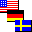All

The Mirror CONCEPT is accomplished by executing the definition of the given call and replacing: EN: 10
Mirror CONCEPT \$B\$O(B, \$BM?\$(\$i\$l\$?%3!<%k\$NDj5A\$rJP: 10
Mirror CONCEPT åstadkoms genom att utföra definitionen på det givna callet och byta ut:  SE: 10
Mirror 概念 是按照定义完成口令但需要: CH: 10

At any point where you would normally pass Right-shoulders, pass Left-shoulders and vice-versa. At any point where you would normally use the Right-hand, use the Left-hand and vice-versa. At any point where the Beaus do something, the Belles do it and vice-versa. EN: 40
Vid något tillfälle där du normalt skall passera med höger axel, passera med vänster axel och vice versa. Vid något tillfälle där du normalt skulle använda höger hand, använd vänster hand och vice versa. Vid något tillfälle där Beaus skall göra något, skall Belles göra det istället och vice versa. SE: 40

 Mirror Swing Thru: Left Swing Thru Mirror Flutter Wheel: Reverse Flutter Wheel Mirror Here Comes The Judge: Sock It To Me Mirror Tag The Line: Pass Left-shoulders instead of Right-shoulders EN: 50 \$B1&8*\$NBe\$o\$j\$K:87?\$GDL\$j2a\$.\$^\$9(B. JP: 50 Passera med vänster axel istället för med höger axel SE: 50 以左肩通过取代右肩通过 CH: 50 AddCzech Mirror Slide Thru: Left Pass Thru; Boys 1/4 Left as Girls 1/4 Right EN: 60 Left Pass Thru \$B\$r\$7(B; Boys 1/4 Left, Girls 1/4 Right JP: 60 Left Pass Thru; Boys 1/4 Left medan Girls 1/4 Right SE: 60 Left Pass Thru; Boys 1/4 Left 同时 Girls 1/4 Right CH: 60 AddCzech Mirror Wheel & Deal: (from a One-Faced Line): The left-side Couple goes in front EN: 70 (One-Faced Line \$B\$+\$i(B): \$B:8B&\$N%+%C%W%k\$,A0\$K\$J\$j\$^\$9(B JP: 70 (från en One-Faced Line): Beau Couple går framför SE: 70 (起始队形为 One-Faced Line): 左边的 Couple 在前(“右包左”) CH: 70 AddCzech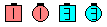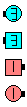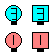before före před Mirror Wheel & Deal\$B\$NA0(B 之前 half-way \$BH>J,\$N\$H\$3\$m(B halvvägs 半途时 after efter po \$B8e(B 之后

When doing the Mirror version of a call in which two dancers end on the same spot (such as an odd Tally-Ho) step to Left hands instead of Right hands! The Mirror CONCEPT is more binding than the Right-hand same-spot rule. EN: 80
Mirror \$B\$G%3!<%k\$r9T\$\$(B, 2 \$B?M\$,F1\$8>l=j\$G=*\$o\$k\$H\$-(B (\$BIaDL\$G\$J\$\$(B Tally-Ho \$B\$N\$h\$&\$J\$H\$-(B), \$B1&Mirror CONCEPT \$B\$OF1\$8>l=j\$G1&JP: 80
När man gör Mirror versionen av ett call där två dansare hamnar på samma position (såsom en udda Tally-Ho) ta vänster hand istället för höger hand! Mirror CONCEPT är överordnat regeln om höger hand vid samma position regeln. SE: 80

Note: The words Left and Reverse have traditionally been used to indicate Mirror (e.g., Left Swing Thru, Reverse Flutter Wheel) but their usage is inconsistent. Dancers may not know what is intended when something like 'Left Circle By' is called. Should one Circle Left the first given fraction then Left Touch the next given fraction? Or, since you are normally moving to the Left on a Circle By, should one Circle Right the first fraction? Reverse can also mean 'In Reverse Order', 'Rewind', or have other meanings ('Reverse Stack The Line': original Trailers instead of Leaders go across to right hands!). If we only had the choice of using Left or Reverse, what would the Mirror version of Shake & Rattle be called? The Mirror version of Shakedown is called Left Shakedown and the Mirror version of Reverse Split Swap is called Split Swap! The Mirror CONCEPT resolves these ambiguities. EN: 90
\$BCmLeft \$B\$H(B Reverse \$B\$,(B Mirror \$B\$r<(\$98@MU\$H\$7\$F;H\$o\$l\$F\$-\$^\$7\$?(B (\$BNc\$(\$P(B, Left Swing Thru, Reverse Flutter Wheel), \$B\$7\$+\$7\$=\$l\$i\$N;H\$\$J}\$K\$O0l4S@-\$,\$"\$j\$^\$;\$s(B. 'Left Circle By' \$B\$N\$h\$&\$J%3!<%k\$,\$J\$5\$l\$?\$H\$-(B, \$B%@%s%5!<\$O2?\$r0U?^\$7\$F\$\$\$k\$N\$+J,\$+\$i\$J\$\$\$G\$7\$g\$&(B. Circle Left \$B\$r;O\$a\$N(B fraction \$B\$@\$19T\$\$(B, Left Touch \$B\$rReverse \$B\$O(B 'In Reverse Order', 'Rewind' \$B\$r0UL#\$7(B, \$B\$^\$?B>\$N0UL#\$N\$3\$H(B ('Reverse Stack The Line': \$B%*%j%8%J%k\$N(B Leaders \$B\$G\$J\$/(B Trailers \$B\$,9T\$-(B\$B1&\$B\$r\$H\$j\$^\$9(B!) \$B\$b\$"\$j\$^\$9(B. \$B\$b\$7(B Left \$B\$+(B Reverse \$B\$N\$I\$A\$i\$+\$r;H\$o\$J\$1\$l\$P\$J\$i\$J\$\$\$H\$7\$?\$i(B, Shake & Rattle \$B\$N(B Mirror \$B\$O\$I\$A\$i\$r;H\$&\$G\$7\$g\$&\$+(B? Shakedown \$B\$N(B Mirror \$B\$O(B Left Shakedown \$B\$H%3!<%k\$5\$l(B, Reverse Split Swap \$B\$N(B Mirror \$B\$O(B Split Swap \$B\$H%3!<%k\$5\$l\$^\$9(B! Mirror CONCEPT \$B\$O\$3\$l\$i\$N[#Kf\$5\$r2r7h\$7\$^\$9(B. JP: 90
\$BCmLeft \$B\$H(B Reverse \$B\$,(B Mirror \$B\$r<(\$98@MU\$H\$7\$F;H\$o\$l\$F\$-\$^\$7\$?(B (\$BNc\$(\$P(B, Left Swing Thru, Reverse Flutter Wheel), \$B\$7\$+\$7\$=\$l\$i\$N;H\$\$J}\$K\$O0l4S@-\$,\$"\$j\$^\$;\$s(B. 'Left Circle By' \$B\$N\$h\$&\$J%3!<%k\$,\$J\$5\$l\$?\$H\$-(B, \$B%@%s%5!<\$O2?\$r0U?^\$7\$F\$\$\$k\$N\$+J,\$+\$i\$J\$\$\$G\$7\$g\$&(B. Circle Left \$B\$r;O\$a\$N(B fraction \$B\$@\$19T\$\$(B, Left Touch \$B\$rReverse \$B\$O(B 'In Reverse Order', 'Rewind' \$B\$r0UL#\$7(B, \$B\$^\$?B>\$N0UL#\$N\$3\$H(B ('Reverse Stack The Line': \$B%*%j%8%J%k\$N(B Leaders \$B\$G\$J\$/(B Trailers \$B\$,9T\$-(B\$B1&\$B\$r\$H\$j\$^\$9(B!) \$B\$b\$"\$j\$^\$9(B. \$B\$b\$7(B Left \$B\$+(B Reverse \$B\$N\$I\$A\$i\$+\$r;H\$o\$J\$1\$l\$P\$J\$i\$J\$\$\$H\$7\$?\$i(B, Shake & Rattle \$B\$N(B Mirror \$B\$O\$I\$A\$i\$r;H\$&\$G\$7\$g\$&\$+(B? Shakedown \$B\$N(B Mirror \$B\$O(B Left Shakedown \$B\$H%3!<%k\$5\$l(B, Reverse Split Swap \$B\$N(B Mirror \$B\$O(B Split Swap \$B\$H%3!<%k\$5\$l\$^\$9(B! Mirror CONCEPT \$B\$O\$3\$l\$i\$N[#Kf\$5\$r2r7h\$7\$^\$9(B. SE: 90
\$BCmLeft \$B\$H(B Reverse \$B\$,(B Mirror \$B\$r<(\$98@MU\$H\$7\$F;H\$o\$l\$F\$-\$^\$7\$?(B (\$BNc\$(\$P(B, Left Swing Thru, Reverse Flutter Wheel), \$B\$7\$+\$7\$=\$l\$i\$N;H\$\$J}\$K\$O0l4S@-\$,\$"\$j\$^\$;\$s(B. 'Left Circle By' \$B\$N\$h\$&\$J%3!<%k\$,\$J\$5\$l\$?\$H\$-(B, \$B%@%s%5!<\$O2?\$r0U?^\$7\$F\$\$\$k\$N\$+J,\$+\$i\$J\$\$\$G\$7\$g\$&(B. Circle Left \$B\$r;O\$a\$N(B fraction \$B\$@\$19T\$\$(B, Left Touch \$B\$rReverse \$B\$O(B 'In Reverse Order', 'Rewind' \$B\$r0UL#\$7(B, \$B\$^\$?B>\$N0UL#\$N\$3\$H(B ('Reverse Stack The Line': \$B%*%j%8%J%k\$N(B Leaders \$B\$G\$J\$/(B Trailers \$B\$,9T\$-(B\$B1&\$B\$r\$H\$j\$^\$9(B!) \$B\$b\$"\$j\$^\$9(B. \$B\$b\$7(B Left \$B\$+(B Reverse \$B\$N\$I\$A\$i\$+\$r;H\$o\$J\$1\$l\$P\$J\$i\$J\$\$\$H\$7\$?\$i(B, Shake & Rattle \$B\$N(B Mirror \$B\$O\$I\$A\$i\$r;H\$&\$G\$7\$g\$&\$+(B? Shakedown \$B\$N(B Mirror \$B\$O(B Left Shakedown \$B\$H%3!<%k\$5\$l(B, Reverse Split Swap \$B\$N(B Mirror \$B\$O(B Split Swap \$B\$H%3!<%k\$5\$l\$^\$9(B! Mirror CONCEPT \$B\$O\$3\$l\$i\$N[#Kf\$5\$r2r7h\$7\$^\$9(B. CH: 90

Hint: If you can imagine dancing in a mirrored room and executing a call normally, your reflection would be dancing the Mirror version of that call. EN: 100
\$B%R%s%H(B: Mirror (\$B6@(B) \$B\$,\$"\$kIt20\$NCf\$K\$\$\$FIaDL\$K%3!<%k\$rF0\$\$\$FMY\$C\$F\$\$\$k\$H\$-(B, \$B6@\$NCf\$G\$O%3!<%k\$r(B Mirror \$B\$GMY\$C\$F\$\$\$^\$9(B. JP: 100
Tips: Om du kan föreställa dig att du dansar i ett rum med speglar och utför ett call normalt, så kommer din spegelbild att dansa Mirror versionen av detta call. SE: 100

Common misconceptions: A Mirror Pass The Ocean is not a Pass The Sea. A Mirror Pass The Ocean involves a Left-shoulder Pass Thru, whereas a Pass The Sea involves a Right-shoulder Pass Thru. EN: 110
\$B6&DL\$N4V0c\$\$(B: Mirror Pass The Ocean \$B\$O(B Pass The Sea \$B\$G\$O\$"\$j\$^\$;\$s(B. Mirror Pass The Ocean \$B\$G\$O:88*\$N(B Pass Thru \$B\$G\$9\$,(B, Pass The Sea \$B\$G\$O1&8*\$N(B Pass Thru \$B\$G\$9(B. JP: 110
Vanliga missuppfattningar: En Mirror Pass The Ocean är inte en Pass The Sea. En Mirror Pass The Ocean har en Pass Thru med vänster axel medan Pass The Sea har en Pass Thru med höger axel. SE: 110

Note: For many calls, the Mirror version of the call is the same as the call itself. For example, a Mirror Scoot Back is a Scoot Back (since the definition of Scoot Back does not involve Beaus|Belles or Left|Right); Similarly, when starting from a Wave, a Mirror Spin The Top is the same as Spin The Top. EN: 120
\$BCmJP: 120
Notera: För många call, är Mirror versionen av callet samma som callet självt. Till exempel, en Mirror Scoot Back är en Scoot Back (eftersom definitionen för Scoot Back inte blandar in Beaus|Belles eller Left|Right); På samma sätt, om man börjar från en Wave, är Mirror Spin The Top samma som Spin The Top. SE: 120

Mirror Circle By fraction By fraction | anything [C3B]:
From Facing Couples. Circle Right the first given fraction and Step To A Left-Hand Wave; Arm Turn the second given fraction or do the anything call. This call has historically been known as Reverse Circle ByEN: 476
\$B8~\$\$9g\$C\$?%+%C%W%k\$+\$i(B. Circle Right \$B\$r;O\$a\$N(B fraction \$B\$@\$19T\$\$(B Step To A L-H Wave \$B\$r\$7(B; Arm Turn \$B\$rfraction \$B\$@\$1\$9\$k\$+\$^\$?\$O(B anything \$B\$N%3!<%k\$r9T\$\$\$^\$9(B. \$B\$3\$N%3!<%k\$N(B fraction \$B\$N%G%U%)%k%H\$O\$"\$j\$^\$;\$s(B. \$BNr;KE*\$K\$O(B Reverse Circle By \$B\$H\$7\$FCN\$i\$l\$F\$\$\$^\$9(B. JP: 476
Aus Facing Couples. Circle Right die erste gegebene fraction und Step To A L-H Wave; Arm Turn die zweite gegebene fraction oder tanze den anything Call. Diese Figur ist historisch auch als Reverse Circle By bekannt. DE: 476
Från Facing Couples. Circle Right den första givna fraction och Step To A Left-Hand Wave; Arm Turn den andra givna fraction eller gör anything callet. Detta call har historiskt varit känt som Reverse Circle BySE: 476
Z formace Facing Couples. Circle Right první fraction a Step To A Left-Hand Wave; Arm Turn druhý fraction nebo proveďte figuru anything. Tato figura je historicky známá jako Reverse Circle ByCZ: 476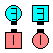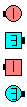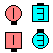before före před Mirror Circle By 1/4 By 1/4\$B\$NA0(B 之前 after efter po Circle Right 1/4 and \$B\$H(B och a 并做 Step To A L-H Wave\$B\$N8e(B 之后 after efter po Arm Turn 1/4\$B\$N8e(B 之后 (done) (\$B=*\$o\$j(B) (klart) (hotovo) (完成)

Mirror Recycle [C3B]:
From Facing Couples. Belles Extend and Left-Face U-Turn Back as Beaus Dodge into the Belle's original starting position and Veer Right. Ends in a L-H Wave. Historically known as Reverse RecycleEN: 782
\$B8~\$\$9g\$C\$?%+%C%W%k\$+\$i(B. Belles \$B\$O(B Extend \$B\$r\$7:82s\$j\$K(B U-Turn Back \$B\$r\$7(B, Beaus \$B\$O(B Belle \$B\$,%3!<%k\$r;O\$a\$k0LCV\$X(B Dodge \$B\$7(B Veer Right \$B\$r\$7\$^\$9(B. \$B:8Reverse Recycle \$B\$H\$7\$FCN\$i\$l\$F\$\$\$^\$9(B. JP: 782
Från Facing Couples. Belles Extend och U-Turn Back åt vänster medan Beaus Dodge till Belles ursrungliga startposition och Veer Right. Slutar i en L-H Wave. Historiskt känt som Reverse RecycleSE: 782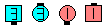before före před Mirror Recycle\$B\$NA0(B 之前 after efter po \$B8e(B 之后

Mystic CONCEPT [C4]:
Center 4 work Mirror as the Outer 4 work normal, and all do the given call. If the call involves the Centers eventually working with the original Outsides, then the completion of the call procedes in a normal manner (i.e., the original Centers no longer work Mirror). In this case, it would be better if the caller said Initially MysticEN: 783
Center 4 \$B\$O(B Mirror \$B\$G(B Outer 4 \$B\$OIaDL\$K(B, \$BM?\$(\$i\$l\$?%3!<%k\$r9T\$\$\$^\$9(B. \$B\$b\$7(B, Centers \$B\$,\$\$\$D\$+%*%j%8%J%k\$N(B Outsides \$B\$HF0\$/\$3\$H\$,\$"\$k%3!<%k\$N\$H\$-\$O(B, \$B\$=\$l0J9_\$OIaDL\$NJ}K!\$G9T\$\$\$^\$9(B (\$B\$9\$J\$o\$A(B, \$B%*%j%8%J%k\$N(B Centers \$B\$O\$=\$l0J>e(B Mirror \$B\$GF0\$-\$^\$;\$s(B). \$B\$3\$N>l9g(B, \$B%3!<%i!<\$O(B Initially Mystic \$B\$H8@\$C\$?J}\$,NI\$\$\$G\$7\$g\$&(B. JP: 783
Centers arbetar Mirror och alla gör det givna callet. Om callet innebär att Centers slutligen arbetar med ursprungliga Outsides, så fortsätter callet på det normala sättet (dvs.,de ursprungliga Centers arbetar inte längre Mirror). I detta fall vore det bättre om callern sa Initially MysticSE: 783Choreography for Mirror ConceptComments? Questions? Suggestions?

https://www.ceder.net/def/_all_languages.php?level=master&action=edit&file=mirror.php
16-December-2019 04:24:37